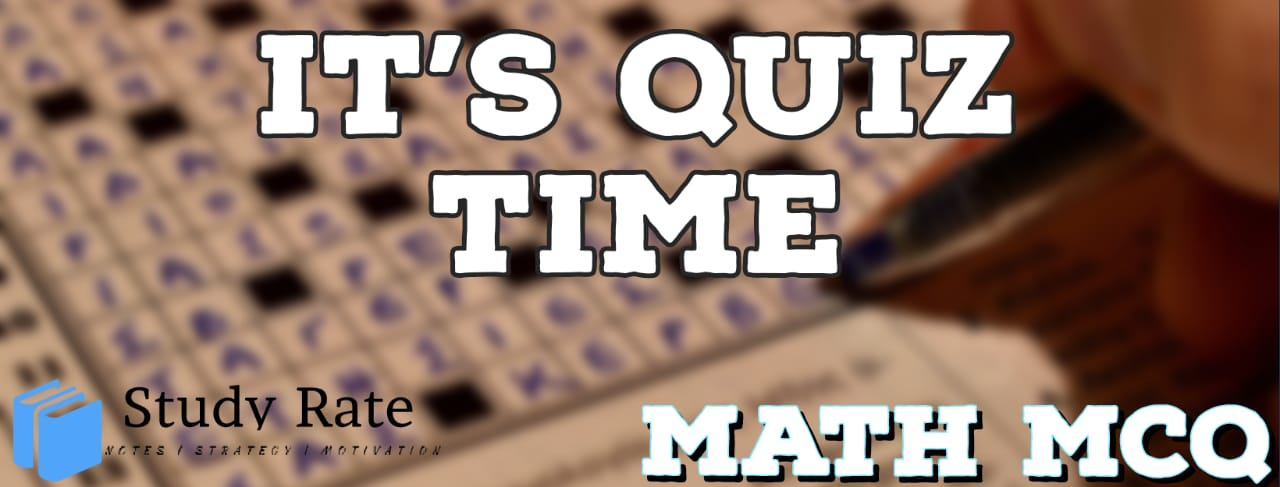## CBSE Class 10 Maths Chapter 5 –Arithmetic Progression MCQ Quiz

Free PDF Download of CBSE Class 10 Maths Chapter 5 Arithmetic Progression Multiple Choice Questions with Answers. MCQ Questions for Class 10 Maths with Answers was Prepared Based on Latest Exam Pattern. Students can solve Class 10 Maths Arithmetic Progression MCQs with Answers to know their preparation level

Arithmetic Progression Class 10 Math Online Quiz MCQ’s

1. Find the number of terms in each of the following APs:
(i) 7,13,19….,205
(ii) 18,31/2,13,…−47

2. Find the 31st term of an AP whose 11th term is 38 and 16th term is 73.

3. Two AP’s have the same common difference. The difference between their 100th terms 100, what is the difference between their 1000th terms.

4. How many three-digit numbers are divisible by 7?

5. The sum of first ten terms of an A.P. is four times the sum of its first five terms, then ratio of the first term and common difference is

6. Find the sum of all the non-negative terms of the following sequence: 100, 97, 94, …

7. The first four terms of an AP, whose first term is –2 and the common difference is –2, are

8. The famous mathematician associated with finding the sum of the first 100 natural
numbers is
(A) Pythagoras (B) Newton
(C) Gauss (D) Euclid

9. If 7 times the 7th term of an AP is equal to 11 times its 11th term, then its 18th term will be

10. Two APs have the same common difference. The first term of one of these is –1 and that of the other is – 8. Then the difference between their 4th terms is